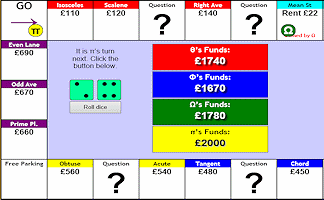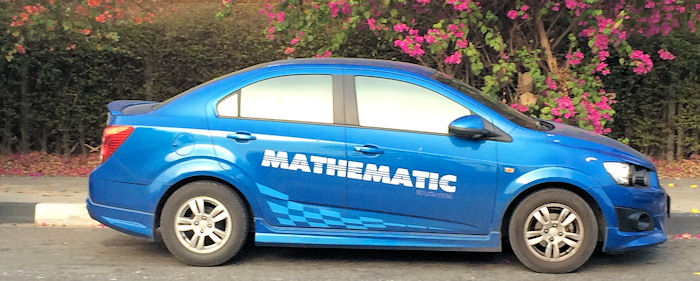### A Maths Starter Of The DayWhat is the total cost of all four cars?

Topics: Starter | Algebra | Problem Solving

• Vijay Leckraz, Dartford Technology College
•
• Let the Blue car be £x. The red car would then be Blue+£5000.Yellow is the same as Blue,so Yellow cost£x.Green cost twice as much as Blue so Green cost £2X.Green car is the same as Red car so we can write
Green car= Red car
2x=x+£50000.
solving this equation by the elimination method we have 2x-x=x-x+£5000
which gives x=£5000
So Blue car costs £5000
Yellow Car costs £5000
Green car costs £10000
Red car costs £10000.
• Jonny,
•
• There's a slight mistake in the answer above:
Green car = Red car
2x=x+£50000.
This should be £5000.
Enjoyed the starter though!
• Phillipa, West Yorkshire
•
• I think this is very good for when Im doing Algebra with Ks3 however can you explain how you did it as we are all baffled.
• Vicki, Cheshire
•
• It's quite simple (when you know how) really... Call the blue car B, yellow car Y, green car G and red car R.
The blue and yellow cars cost the same so B=Y
The green and red cost the same so G=R
R = B + 5,000 and since G=R you can say G = B + 5,000
G = 2Y and since Y=B you can say G = 2B
So make these equal to each other:
2B = B + 5,000
B = 5,000 = Y
G = R = 5,000 + 5,000 = 10,000
The cars total up to 30,000.
• Billy, Newcastle
•
• This question is perfect for drawing using bars. I liked it.

How did you use this starter? Can you suggest how teachers could present or develop this resource? Do you have any comments? It is always useful to receive feedback and helps make this free resource even more useful for Maths teachers anywhere in the world.

Previous Day | This starter is for 16 July | Next Day

Your access to the majority of the Transum resources continues to be free but you can help support the continued growth of the website by doing your Amazon shopping using the links on this page. Below is an Amazon search box and some items I have chosen and recommend to get you started. As an Amazon Associate I earn a small amount from qualifying purchases which helps pay for the upkeep of this website.Teacher, do your students have access to computers?Do they have iPads or Laptops in Lessons? Whether your students each have a TabletPC, a Surface or a Mac, this activity lends itself to eLearning (Engaged Learning).Transum.org/go/?Start=July16

Here is the URL which will take them to game involving the use of money.

Transum.org/go/?to=Mathopoly## Extension

The car pictured below costs three quarters of its cost plus £4500. How much does it cost?